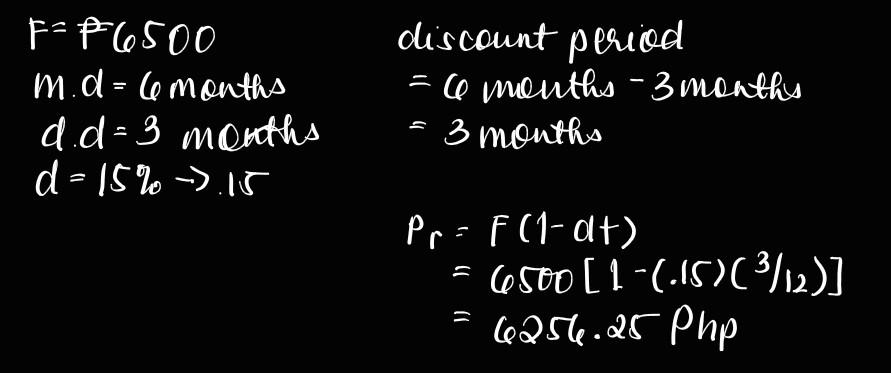# Question Solved1 AnswerIs my answer and solution correct? If not, why? Thank you! I followed the process for a Bank Discount Note (BDN) as taught in class: 1. determine discount period 2. determine proceeds Problem: What is the proceeds of a $6,500 bank discount note that is payable at the end of 6 months but discounted after 3 months at 15% discount interest rate? Solution: F-F6500 m.d = 6 months d.d - 3 months d = 15% -).15 discount period - co months - 3 months 3 months . Pra F (1-dt) 6500[1-(.15)(3/12)] 6256.25 PhpJUZPA7 The Asker · Accounting Is my answer and solution correct? If not, why? Thank you! I followed the process for a Bank Discount Note (BDN) as taught in class: 1. determine discount period 2. determine proceeds Problem: What is the proceeds of a$6,500 bank discount note that is payable at the end of 6 months but discounted after 3 months at 15% discount interest rate?

Solution:Transcribed Image Text: F-F6500 m.d = 6 months d.d - 3 months d = 15% -).15 discount period - co months - 3 months 3 months . Pra F (1-dt) 6500[1-(.15)(3/12)] 6256.25 Php
More
Transcribed Image Text: F-F6500 m.d = 6 months d.d - 3 months d = 15% -).15 discount period - co months - 3 months 3 months . Pra F (1-dt) 6500[1-(.15)(3/12)] 6256.25 Php